# Spbctf winter meetup. My tasks writeups @ Rakovsky Stanislav | Saturday, Jan 30, 2021 | 2 minutes read | Update at Saturday, Jan 30, 2021

I’ve written three tasks for my speech on spbctf winter meetup 2020. Here are writeups on them.

You can watch the writeup on YouTube (on russian).

(open the post to view more)

Writeup status: In progress! Need to catch some time to describe it in English completely. You can see some main points on the pictures below. If you have some questions - contact me)

### Odna pika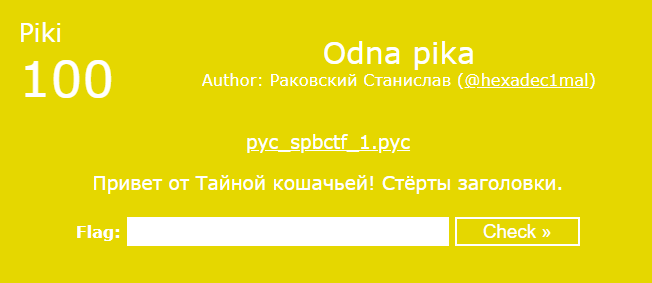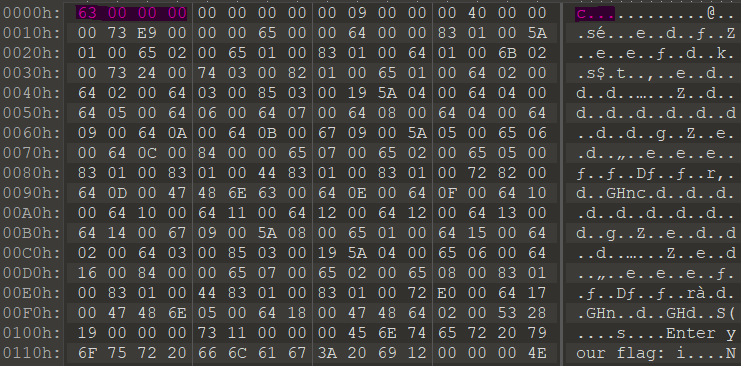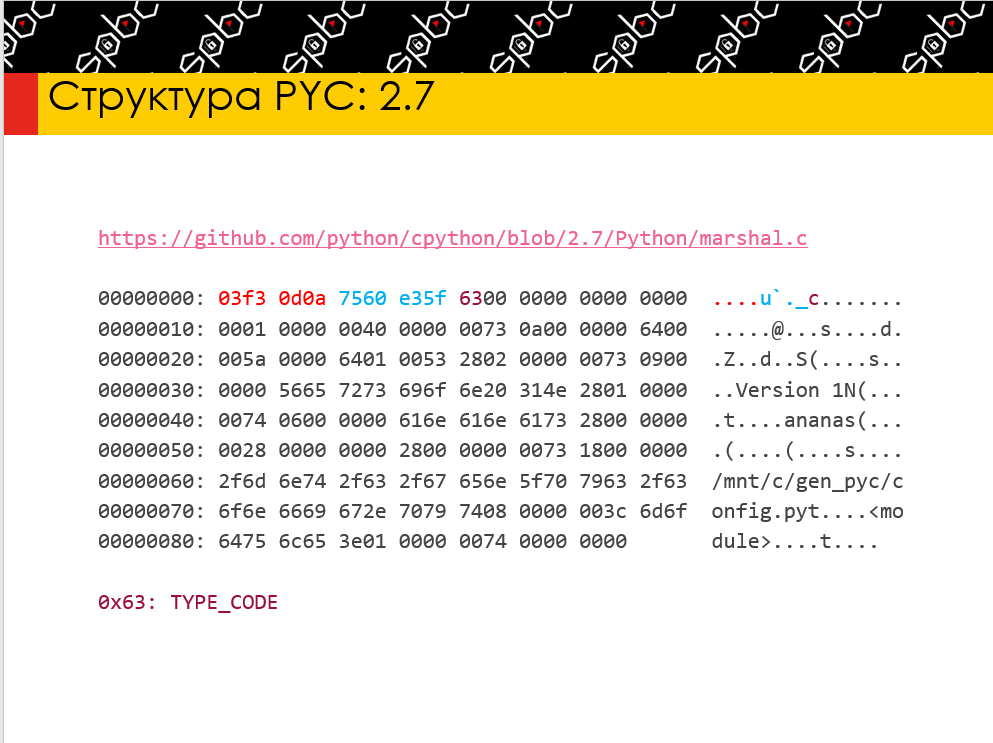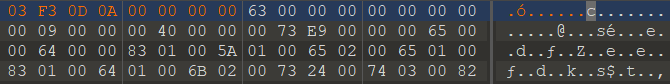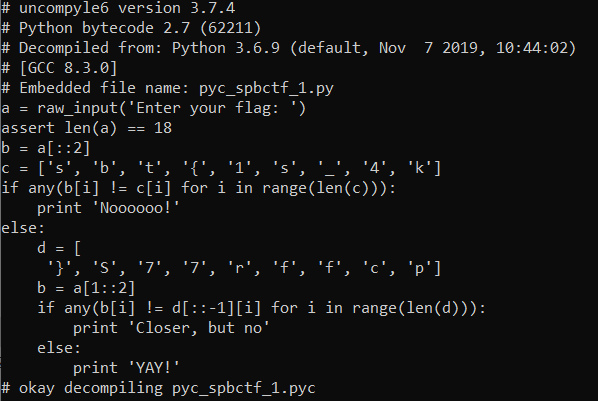``````>>> a = ['s', 'b', 't', '{', '1', 's', '_', '4', 'k']
>>> b = ['}', 'S', '7', '7', 'r', 'f', 'f', 'c', 'p'][::-1]
>>> "".join(a[i] + b[i] for i in range(len(a)))
'spbctf{f1rs7_74Sk}'
``````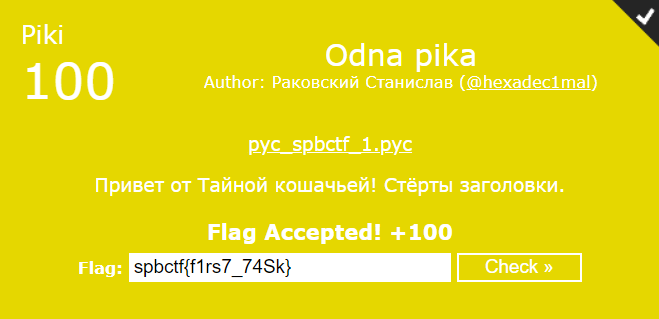### Dve piki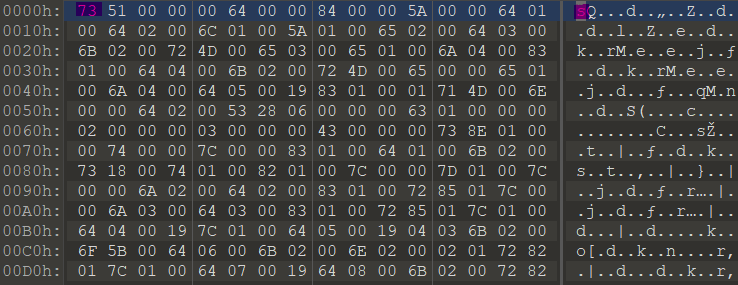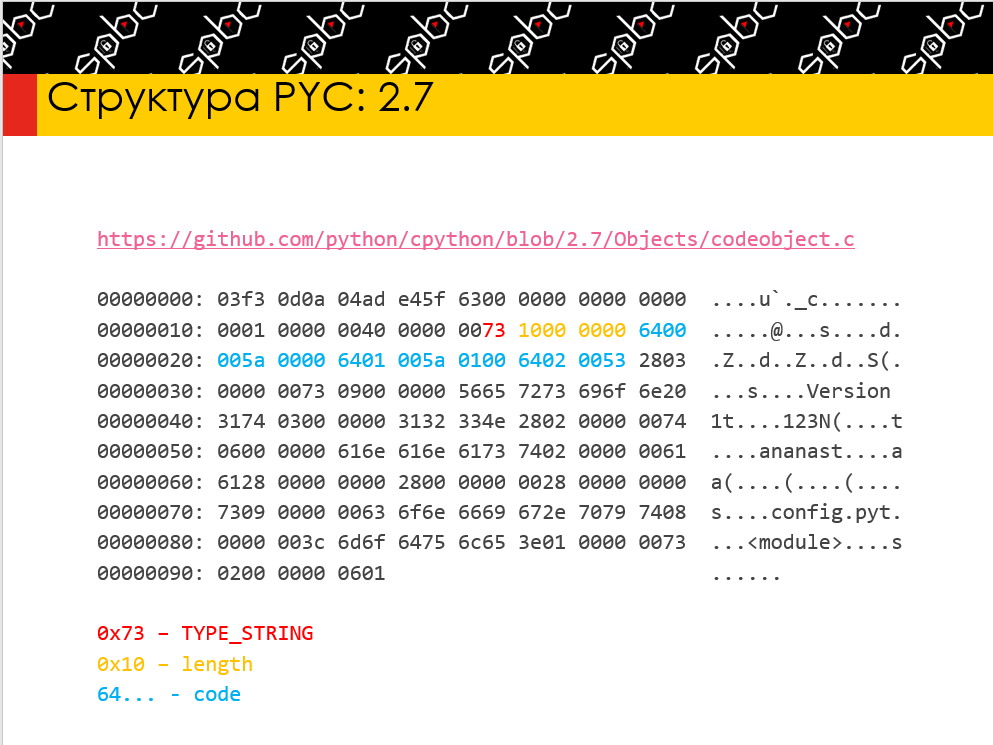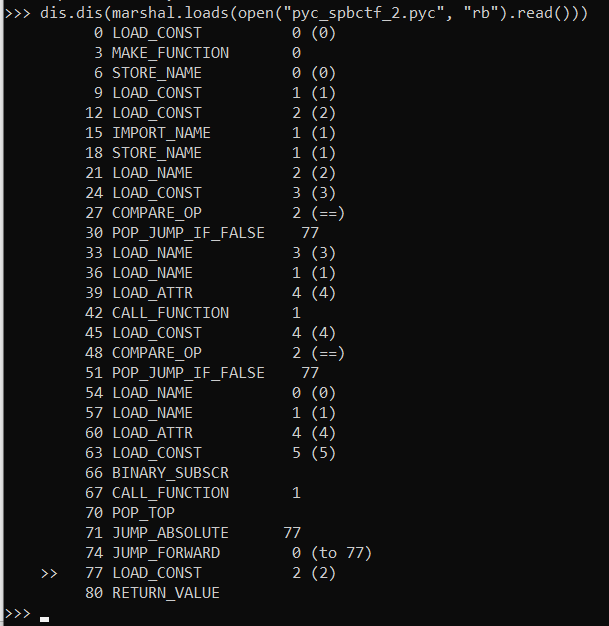``````>>> a = marshal.loads(open("pyc_spbctf_2.pyc", "rb").read())
(<code object spbctf at 0x7f71951036b0, file "pyc_spbctf_2.py", line 3>, -1, None, '__main__', 2, 1)
``````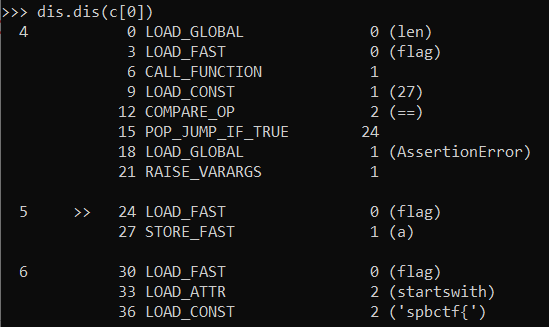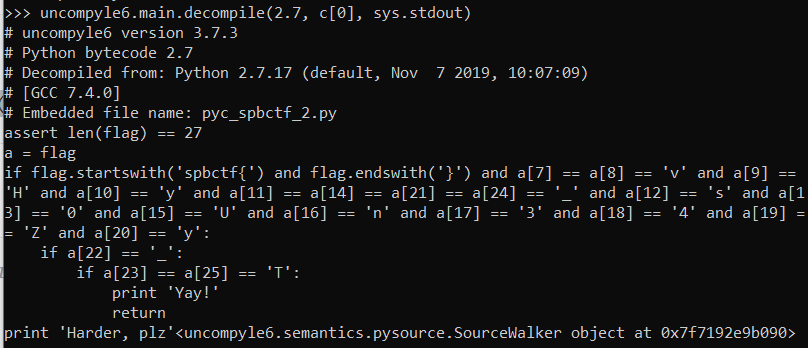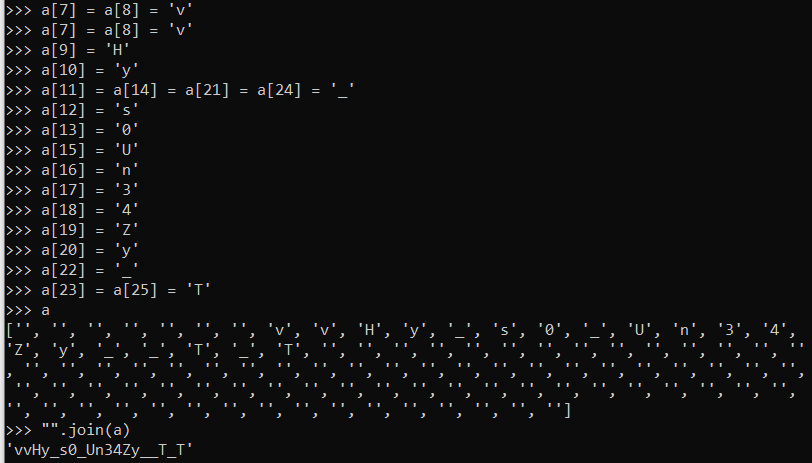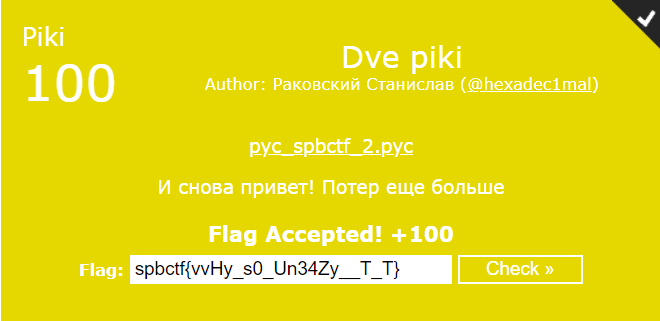### Tri piki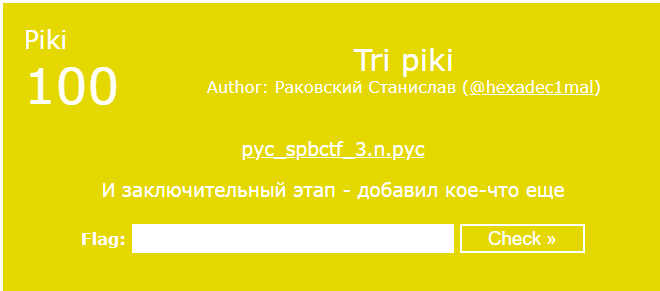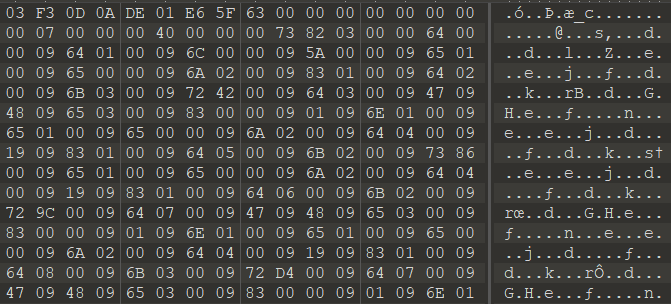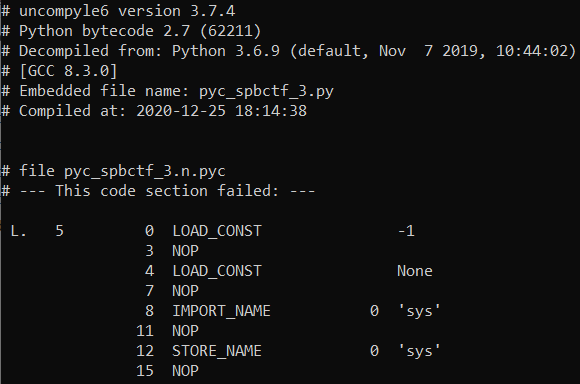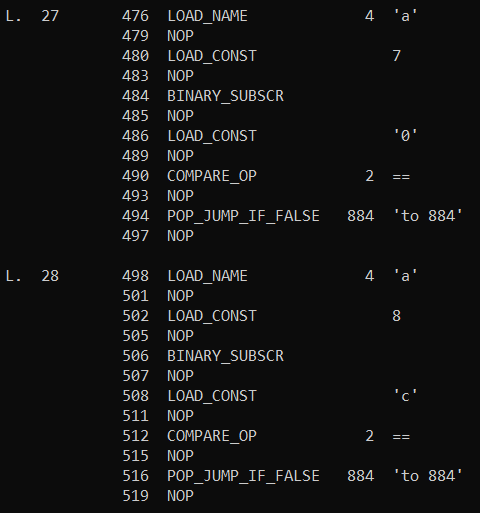``````>>> import bytecode_graph
>>> import marshal
>>> import sys, uncompyle6
>>> from dis import opmap
>>> nodes = [x for x in bcg.nodes()]
>>> for n in nodes:
...  if n.opcode == opmap['NOP']:
...   bcg.delete_node(n)
...
>>> new_code = bcg.get_code()
>>> new_code
<code object <module> at 0x7f5c90502bb0, file "pyc_spbctf_3.py", line 4>
>>> uncompyle6.main.decompile(2.7, new_code, sys.stdout)
# uncompyle6 version 3.7.3
# Python bytecode 2.7
# Decompiled from: Python 2.7.17 (default, Nov  7 2019, 10:07:09)
# [GCC 7.4.0]
# Embedded file name: pyc_spbctf_3.py
import sys
if len(sys.argv) != 2:
print 'Bye!'
exit()
if len(sys.argv) == 25 or len(sys.argv) == 27:
print 'Bye-bye!'
exit()
if len(sys.argv) != 26:
print 'Bye-bye!'
exit()
a = sys.argv
if not all([a == 's',
a == 'p',
a == 'b',
a == 'c',
a == 't' and a == 'f' and a == '{']):
print 'Wow, but not'
exit()
if any([a != '}', a != 'k']):
print 'Bruh'
exit()
if a == '0' and a == 'c' and a == 'h' and a == 'e' and a == 'n' and a == '_' and a == 'z' and a == 'l' and a == '0' and a == 'j' and a == 'n' and a == '1' and a == 'j' and a == '_' and a == '7' and a == '4' and a == 's' and a == 'k':
print 'Great!'
else:
print 'Ahhh'<uncompyle6.semantics.pysource.SourceWalker object at 0x7f5c904c97d0>

``````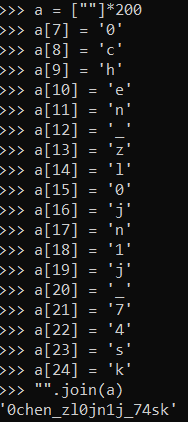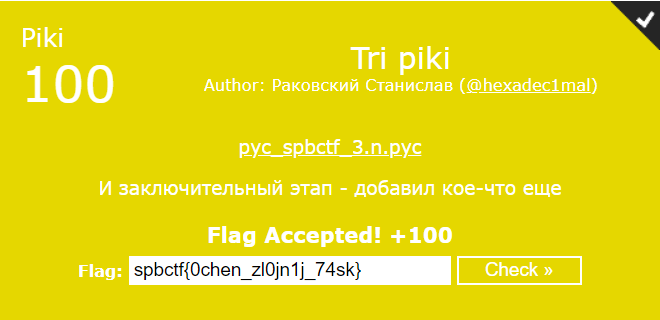### Cats!

Under construction

### Wow! Flipable!

Hello from another side of the Moon!

Looking for a flag? Okay, take this:
LFXXKIDBOJSSA43XMVSXIIC6LYQCAIA=